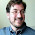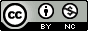## Monday, December 6, 2010

### Using the "Divide by 4 Rule" to Interpret Logistic Regression Coefficients

I was recently reading a bit about logistic regression in Gelman and Hill's book on hierarchical/multilevel modeling when I first learned about the "divide by 4 rule" for quickly interpreting coefficients in a logistic regression model in terms of the predicted probabilities of the outcome. The idea is pretty simple. The logistic curve (predicted probabilities) is steepest at the center where a+ßx=0, where logit-1(x+ßx)=0.5. See the plot below (or use the R code to plot it yourself).

The slope of this curve (1st derivative of the logistic curve) is maximized at a+ßx=0, where it takes on the value:

ße0/(1+e0

=ß(1)/(1+1)²

=ß/4

So you can take the logistic regression coefficients (not including the intercept) and divide them by 4 to get an upper bound of the predictive difference in probability of the outcome y=1 per unit increase in x. This approximation the best at the midpoint of x where predicted probabilities are close to 0.5, which is where most of the data will lie anyhow.

So if your regression coefficient is 0.8, a rough approximation using the ß/4 rule is that a 1 unit increase in x results in about a 0.8/4=0.2, or 20% increase in the probability of y=1.

1.Sweet, thanks Stephen.

2.Very nice!

3.This comment has been removed by the author.

4.Hmmm. I don't trust this. I can see several problems, offhand.

First of all, I believe the 0.20 ( = 0.8/4) in your example is not a 20% change in the probability, but a 0.20 absolute change in the probability. The shortcut thus can easily suggest changes that lead to a probability greater than 1-- for example if the probability when all x=0 is greater than 0.8 in your example.

Second, I don't think you can be sure that most of the data is where p-hat=0.5, unless you mostly have continuous, non-skewed covariates, plus a marginal probability of 0.5. No? Often in practice, the value x=0 is way outside the range of the data, though I guess you could center it.

Even if the marginal probability is 0.5, if you have a dichotomous covariate, it's very unclear what the "upper bound" is in relation to-- there's only one value here. (Maybe if you coded the categories as -0.5, 0.5, this would be a decent approximation, when the marginal probability is 0.5?)

Finally, though, the approach has the same fatal flaw as the odds ratio does, or as risk ratio does if you use the log link instead of the logit link. Namely, it suggests that you can find a useful single number that shows the impact of the covariate. Instead, the import of a covariate with beta = 0.8 varies, depending on the values assigned to the other covariates. Finding a single predicted probability effect this way is as chimerical as finding the risk ratio from the odds ratio-- if the logit link fits the data, we should conclude that there's no fixed risk ratio, i.e., that the log log link does not fit the data. Similarly, if the logit link fits the data, we should conclude that the identity link does not, and that there's no fixed probability-scale effect. I don't think it helps to have a shortcut that implies there is one.

5.A few points:

* Are you talking about Gelman and Hill's book? Might as well give them (or whoever) credit ...

* it's incredibly picky, but it bothers me when people use the "ess-zett" (German double-ess character) rather than beta ...

* @Ken Kleinman: this is an *approximation* that is valid for small values of beta, or small displacements, or both. Obviously if beta=100 it's not very meaningful to say that a 1-unit increase in the predictor will correspond to a 2500% increase in probability ... but it would be meaningful to say that a 0.01-unit increase would correspond to approx. a 25% increase in probability. "x=0" is not a special point here -- it could just as well have been beta_0, where beta_0 is the intercept term.
My personal view is that shortcuts are fine as long as you know their limitations.
Gelman and Hill's book really is very clear and rigorous -- maybe you would agree with their presentation?

6.oops, I see you did link to G&H's book on Amazon. Scratch my point #1.

7.OK, it's an approximation. Is it any help? Using the example code above:

> y[(seq(0,10) * 100) +1]
 0.006692851 0.017986210 0.047425873 0.119202922 0.268941421 0.500000000 0.731058579 0.880797078 0.952574127 0.982013790 0.993307149

So, around x=0, it's pretty close. But if x = 2, a 1-unit increase merits a 0.07 increase in probability. In this this simple setting with one continuous predictor. I think I didn't learn very much from the approximation. But I'll read G&H when I get back to my office.

8.If someone (say a clinician) comes to you and says "hey, the parameter in this logistic regression is 0.01, what the hell does that mean?", this represents one quick, approximate way of telling them how big the effect is *near the midpoint*. If they're more interested in scenarios where the probabilities are near 0 or 1, you could give them the rules of thumb for interpreting log(beta*x) or log(beta*(1-x)) ... if you're going to work with that person for a while, you could spend an hour sitting down and educating them more thoroughly about logit scales.
It may be that you're just not the target audience for this shortcut.

9.Well, I'm certainly not the target audience.

After looking at G&H, my objections still hold, and I think you'd do a disservice to your clinician client if you use the rule of 4 without checking to see if it's meaningful. To see how bad it can be, consider a setting where beta0 = -4, beta1 = 1, and x has a standard normal distribution. (The marginal probability of y is about 0.03.)

The estimated beta1 will be about 1, so the rule of four says that differences of one unit is x can be associated with probability changes no bigger than 0.25. That's great, it's absolutely true, and I have no issue with it. But this applies to values of x that are more than 4 standard deviations above the mean! It's true, but it's totally irrelevant.

I think you're much better off taking three minutes to plot the predicted probs in the data set. Give the plot to the client, maybe. Report a linear approximation, if you don't have time to educate your client. In this case, you could report that a one-unit change is associated with about a change of 0.04 in the probability of the outcome. Or even that at the upper extreme of the data it's associated with a change of about 0.1. I think it's better to get the client to see you as an educator and a resource, so I usually invest the time. That brings the client back and helps establish your credibility.

Note that it is _often_ the case that the marginal probabilities are small, and in fact many epidemiologists distrust logistic regression when the baseline probability is "large" (by which they mean 0.2 or so) because their received wisdom is that in such cases the OR no longer resembles the RR.